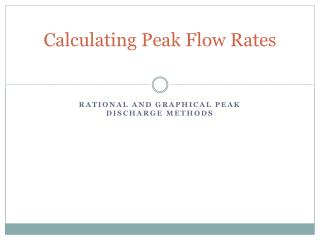DownloadDownload PresentationCalculating Peak Flow Rates

# Calculating Peak Flow Rates

Télécharger la présentation## Calculating Peak Flow Rates

- - - - - - - - - - - - - - - - - - - - - - - - - - - E N D - - - - - - - - - - - - - - - - - - - - - - - - - - -
##### Presentation Transcript

1. Calculating Peak Flow Rates Rational and graphical peak discharge methods

2. Rational Method • Empirical method developed in 1851 to estimate qp (cfs) • Many limitations but still widely used • qp = peak flow (cfs) • C = coefficient (dimensionless) • i = rainfall intensity (in/hr) with duration (D) = tc • tc = time of concentration • A = drainage area (ac) • 1.008 is conversion factor from ac-in/hr to cfs.

3. Rational Method • Rationale of rational method: • As rain falls steadily across watershed runoff will increase until the entire watershed is contributing runoff • Occurs when rainfall duration (D) = tc • If D ≠ tc , runoff less than when D = tc • qp is at a maximum when D = tc • Assumptions of rational method: • Uniform rainfall over drainage area • Maximum qp is a function of the average rain intensity • Runoff frequency = rainfall frequency used

4. Time of Concentration (tc) • The time it takes flow to move from the most hydraulically remote point in a watershed to the watershed outlet • The distance from the hydraulically most remote point to the outlet is called the hydraulic length • tc is the sum of flow times for the various flow segments as the water travels to the watershed outlet • Overland flow + shallow channel flow + open channel flow • Travel time for each segment depends on length of travel and flow velocity

5. Hydraulically most remote point in the watershed

6. Rational Method • Runoff Coefficient (C) • Difficult to accurately determine • Must reflect factors such as: interception, infiltration, surface detention, antecedent moisture conditions • Studies have shown that C is not a constant • C increases with wetter conditions • Table 5.5 contains a range of values for C • Compute average C for composite areas • Area weighted basis • Cavg = SCiAi / SAi(same method used with Curve Numbers)

7. Runoff Coefficients for the Rational Method

8. Rational Method Summary • Peak flow equation only • Cannot predict time to the peak (tp) • Should not be used to develop hydrographs

9. Example Problem Given: • Location: St. Louis, MO • Watershed area: 40 ha (1 ha = 2.47 ac) • 30 ha, medium density residential / 7% slope / HSG = A • 3 ha, high density residential / 1% slope / HSG = C • 7 ha , agricultural / 4% slope / HSG = B • tc = 60 min. • Rainfall event: 5-yr storm • Required: • Find the peak runoff (qp)

10. Example Problem Solution: • Rainfall intensity (i) = 2.0 iph (Figure 2.17) • Land Use Area Area C (ha) (%) (Table 5.3) Med. Residential 30 75.0 0.31 High Residential 3 7.5 0.49 Agricultural 7 17.5 0.15 • Cavg = 0.31(0.75) + 0.49(0.075) + 0.15(0.175) = 0.30 • Area = 40 ha = 98.8 ac • qp = 1.008CiA = 0.30(2.0 in./hr)98.8 ac  qp = 59.75 cfs

11. AGSM 335 • Homework # 5

12. Time of concentration calculation • Most common tc equation for use with Rational Method. • tc – time of concentration (minutes) • L – hydraulic length (ft) • S – slope along hydraulic length (ft/ft)

13. Graphical Peak Discharge Method • Used for small rural and urban watersheds • q – peak discharge (ft3/s) • qu – unit peak discharge (ft3/s-mile-in) [Fig. 5.16 and 5.17; based on Ia = 0.2S and tc] • A – drainage area (mi2) • Q – runoff depth based on 24 hour rainfall (in) [Curve Number Q] • F – adjustment factor for ponds and swamps. [Table 5.3]

14. tc for Graphical Peak Discharge Method • Based on NRCS lag equation: • tL – lag time (hours) • L – hydraulic length (ft) • S – CN (II) S • Y - average land slope (%) • Time of concentration (tc)

15. tc for Graphical Peak Discharge Method • If the CN conditions for Eqn. (5.6) not met than can use a combination of Eqns. (5.8, 5.9 and 5.10) or • v = velocity (ft/s) • K2 is from Table 5.4 • S = slope (%) • tc – time of concentration (hrs)

16. BAEN 460 • Homework #5